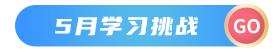## 函数式编程的概念

• 纯函数：这些函数有两个主要属性。首先，它们总是为相同的参数产生相同的输出，而不管其他任何事情。其次，它们没有副作用，即它们确实修改了任何参数或全局变量或输出了一些东西。
• 递归：函数式语言中没有“for”或“while”循环。函数式语言中的迭代是通过递归实现的。
• 函数是一等的并且可以是高阶的：一等函数被视为一等变量。第一类变量可以作为参数传递给函数，可以从函数返回或存储在数据结构中。
• 变量是不可变的：在函数式编程中，我们不能在变量初始化后对其进行修改。我们可以创建新变量——但我们不能修改现有变量。

## Python 中的函数式编程

Python 也支持函数式编程范式，而无需任何特殊功能或库的支持。

### 纯函数

• 它总是为相同的参数产生相同的输出。例如，无论如何，3+7 永远是 10。
• 它不会更改或修改输入变量。

``````# 演示纯函数的 Python 程序

# 一个不改变输入列表并返回新列表的纯函数
def pure_func(List):

New_List = []

for i in List:
New_List.append(i**2)

return New_List

# 驱动程序代码
Original_List = [1, 2, 3, 4]
Modified_List = pure_func(Original_List)

print("Original List:", Original_List)
print("Modified List:", Modified_List)
``````

``````Original List: [1, 2, 3, 4]
Modified List: [1, 4, 9, 16]
``````

### 递归

``````# 演示递归的 Python 程序

# 递归函数查找列表的总和
def Sum(L, i, n, count):

# Base case
if n <= i:
return count

count += L[i]

# 进入递归
count = Sum(L, i + 1, n, count)

return count

# 驱动程序代码
L = [1, 2, 3, 4, 5]
count = 0
n = len(L)
print(Sum(L, 0, n, count))
``````

``````15
``````

### 函数是一等的，可以是高阶的

• 函数是 Object 类型的实例。
• 你可以将函数存储在变量中。
• 你可以将函数作为参数传递给另一个函数。
• 你可以从函数返回函数。
• 你可以将它们存储在数据结构中，例如哈希表、列表……
``````# 演示高阶函数的 Python 程序

def shout(text):
return text.upper()

def whisper(text):
return text.lower()

def greet(func):
# 将函数存储在变量中
greeting = func("我是由作为参数传递的函数创建的。")
print(greeting)

greet(shout)
greet(whisper)
``````

``````我是由作为参数传递的函数创建的。

``````

### Map()

map() 函数在将给定函数应用于给定迭代（列表、元组等）的每个项目后返回结果列表

fun：这是一个函数，map 将给定迭代的每个元素传递给该函数。
iter：它是一个要被映射的可迭代对象。

``````# 用于演示 map 工作的 Python 程序。

# Return double of n
return n + n

# 我们使用 map() 将所有数字翻倍
numbers = (1, 2, 3, 4)

# 不打印值
print(results)

# 打印值
for result in results:
print(result, end = " ")
``````

``````<map object at 0x7fae3004b630>
2 4 6 8
``````

### filter()

filter() 方法在一个函数的帮助下过滤给定的序列，该函数测试序列中的每个元素是否为真。

function：测试序列的每个元素是否为真的函数。
sequence：需要过滤的序列，可以是集合、列表、元组或任何迭代器的容器。

``````# 演示过滤器工作的 Python 程序。

# 过滤元音的函数
def fun(variable):

letters = ['a', 'e', 'i', 'o', 'u']

if (variable in letters):
return True
else:
return False

# sequence
sequence = ['g', 'e', 'e', 'j', 'k', 's', 'p', 'r']

# 使用过滤功能
filtered = filter(fun, sequence)

print('过滤后的字母是：')

for s in filtered:
print(s)
``````

``````过滤后的字母是：
e
e
``````

### Lambda 函数

lambda 参数：表达式

1. 这个函数可以有任意数量的参数，但只有一个表达式，它被计算并返回。
2. 任何需要函数对象的地方都可以使用 lambda 函数。
3. 你需要了解 lambda 函数在语法上仅限于单个表达式。
4. 除了函数中的其他类型的表达式外，它在特定的编程领域有多种用途。

``````# 演示 lambda 的 Python 代码

cube = lambda x: x * x*x
print(cube(7))

L = [1, 3, 2, 4, 5, 6]
is_even = [x for x in L if x % 2 == 0]

print(is_even)
``````

``````343
[2, 4, 6]
``````

### 生成器

#### 生成器函数

``````# 一个生成器函数，第一次产生 1，第二次产生 2，第三次产生 3
def simpleGeneratorFun():
yield 1
yield 2
yield 3

# 用于检查上述生成器功能的驱动代码
for value in simpleGeneratorFun():
print(value)
``````

``````1
2
3
``````

#### 生成器对象

``````# 一个 Python 程序，用于演示通过 next() 使用生成器对象

# 一个生成器函数
def simpleGeneratorFun():
yield 1
yield 2
yield 3

# x 是一个生成器对象
x = simpleGeneratorFun()

# 使用 next 遍历生成器对象
print(x.next()) # In Python 3, __next__()
print(x.next())
print(x.next())
``````

``````1
2
3
``````

``````# 斐波那契数列的简单生成器
def fib(limit):

# 初始化前两个斐波那契数
a, b = 0, 1

# 一个接一个地生成下一个斐波那契数
while a < limit:
yield a
a, b = b, a + b

# 创建一个生成器对象
x = fib(5)

# 使用 next 遍历生成器对象
print(x.next()) # In Python 3, __next__()
print(x.next())
print(x.next())
print(x.next())
print(x.next())

# 使用 for in 循环遍历生成器对象。
print("\n在循环中使用 for")
for i in fib(5):
print(i)
``````

``````0
1
1
2
3

0
1
1
2
3
``````

### 装饰器

``````@hy_decorator
def hello_decorator():
print("HY")

# 上面的代码等价于 -

def hello_decorator():
print("HY")

hello_decorator = hy_decorator(hello_decorator)'''
``````

``````# 定义装饰器
def hello_decorator(func):

# inner1 是一个 Wrapper 函数，其中调用了参数

# 内部函数可以访问外部局部函数，如本例中的“func”
def inner1():
print("这是在函数执行之前")

# 现在在包装函数中调用实际函数。
func()

print("这是在函数执行之后")

return inner1

# 定义一个函数，在包装器内部调用
def function_to_be_used():
print("这是在函数内部")

# 在装饰器内部传递“function_to_be_used”来控制其行为
function_to_be_used = hello_decorator(function_to_be_used)

# 调用函数
function_to_be_used()
``````

``````这是在函数执行之前

``````

``````# 导入库
import time
import math

# 装饰器来计算任何函数的持续时间
def calculate_time(func):

# 在 inner1 中添加的参数，如果函数接受任何参数，可以像这样添加。
def inner1(*args, **kwargs):

# 在函数执行前存储时间
begin = time.time()

func(*args, **kwargs)

# 函数执行后存储时间
end = time.time()
print("总时间：", func.__name__, end - begin)

return inner1

# 这可以添加到任何存在的函数中，在这种情况下计算阶乘
@calculate_time
def factorial(num):

# 因为它花费的时间非常少，因此 sleep 2 秒你可以看到实际的差异
time.sleep(2)
print(math.factorial(num))

# 调用函数
factorial(10)
``````

``````3628800

``````

### 不变性

``````# 演示不可变数据类型的 Python 程序

# 字符串数据类型
immutable = "Haiyong"

# 更改值将引发错误
immutable = 'K'
``````

``````Traceback (most recent call last):
File "/home/ee8bf8d8f560b97c7ec0ef080a077879.py", line 10, in
immutable = 'K'
TypeError: 'str' object does not support item assignment
``````